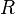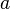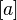# Equivalence relation facts for kids

Kids Encyclopedia Facts

In mathematics, an equivalence relation$R$ on a set is a mathematical relation that is symmetric, transitive and reflexive. For a given element$a$ on that set, the set of all elements related to$a$ (in the sense of$R$) is called the equivalence class of$a$, and written as$[a]$.

With an equivalence relation, it is possible to partition a set into distinct equivalence classes. As an example, consider the set of all animals on a farm and define the following relation: two animals are related if they belong to the same species. Under this relation, a cow is related to an ox, but not to a chicken. In fact, this relation is an equivalence relation because:

• It is reflexive: each animal is of the same species as itself
• It is symmetric: if a first animal is in the same species as a second animal, then the second animal is also in the same species as the first animal.
• It is transitive: if a first animal is in the same species as a second animal, and the second animal is in the same species as a third animal, then the first animal is in the same species as the third animal.

In this example, the set of all animals related to a particular ox forms an equivalence class—it is the set of cattle. In fact, the set of all animals on this farm can be partitioned into different equivalence classes (in this case species).

## Related pagesEquivalence relation Facts for Kids. Kiddle Encyclopedia.